# Taylor variance decomposition¶

The Taylor variance decomposition (also referred as quadratic cumul method or perturbation method) is a probabilistic approach designed to propagate the uncertainties of the input variables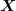through the model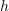towards the output variables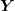. It enables to access the central dispersion (expectation and variance) of the output variables.
This method is based on a Taylor decomposition of the output variabletowards therandom vectors around the mean point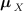. Depending on the order of the Taylor decomposition (classically first order or second order), one can obtain different formulas. For easiness of the reading, we first present the formulas with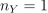before the ones obtained for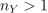.
As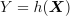, the Taylor decomposition around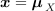at the second order yields to:
Case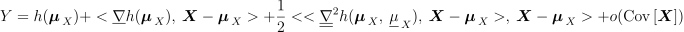where:

•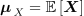is the vector of the input variables at the mean values of each component.

•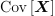is the covariance matrix of the random vector. The elements are the followings :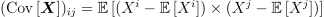•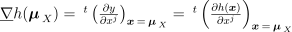is the gradient vector taken at the valueand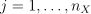.

•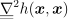is a matrix. It is composed by the second order derivative of the output variable towards the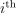and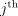components oftaken around. It yields to: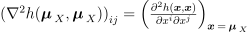•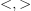is a scalar product between two vectors.

Approximation at the order 1 - Case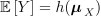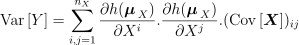Approximation at the order 2 - Case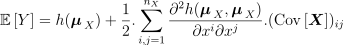The decomposition of the variance at the order 2 is not implemented. It requires both the knowledge of higher order derivatives of the model and the knowledge of moments of order strictly greater than 2 of the pdf.
CaseThe perturbation approach can be developed at different orders from the Taylor decomposition of the random vector. As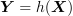, the Taylor decomposition aroundat the second order yields to: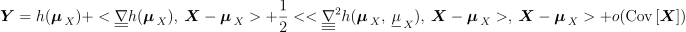where:

•is the vector of the input variables at the mean values of each component.

•is the covariance matrix of the random vector. The elements are the followings :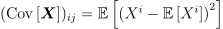•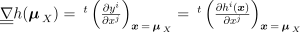is the transposed Jacobian matrix with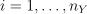and.

•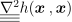is a tensor of order 3. It is composed by the second order derivative towards theandcomponents ofof the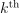component of the output vector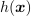. It yields to: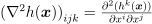•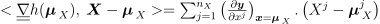•Approximation at the order 1 - Case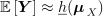Pay attention that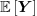is a vector. Thecomponent of this vector is equal to thecomponent of the output vector computed by the modelat the mean value.is thus the computation of the model at mean.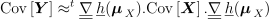Approximation at the order 2 - Case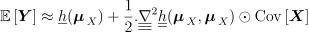This last formulation is the reduced writing of the following expression: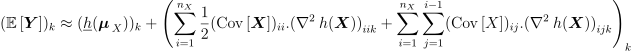The decomposition of the variance at the order 2 is not implemented. It requires both the knowledge of higher order derivatives of the model and the knowledge of moments of order strictly greater than 2 of the pdf.